Author: Oscar Cronquist Article last updated on August 21, 2020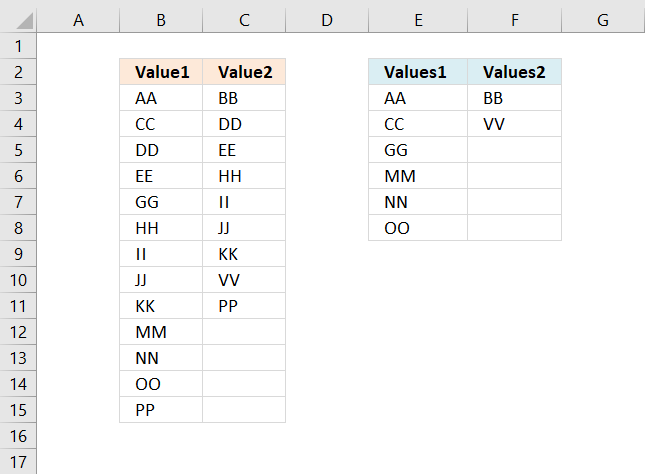This article demonstrates a formula that extracts values that exist only in one column out of two columns.

There are text values in column B and column C.

##### Update!

Excel 365 formula in cell E3:

=INDEX(\$B\$3:\$B\$15, SMALL(IF(COUNTIF(\$C\$3:\$C\$11, \$B\$3:\$B\$15)=0, SEQUENCE(ROWS(\$B\$3:\$B\$15)), ""), ROWS(\$A\$1:A1)))

Excel 365 formula in cell F3:

=INDEX(\$C\$3:\$C\$11, SMALL(IF(COUNTIF(\$B\$3:\$B\$15, \$C\$3:\$C\$11)=0, SEQUENCE(ROWS(\$C\$3:\$C\$11)), ""), ROWS(\$A\$1:A1)))

The formulas above are entered like regular formulas. They contain the SEQUENCE function that older Excel versions are missing.

Copy cell E3 and F3 and paste to cells below as far as needed.

##### Array formula for older Excel versions

The array formula in cell E3 extracts values existing only in column B, compared to column C:

=INDEX(\$B\$3:\$B\$15, SMALL(IF(COUNTIF(\$C\$3:\$C\$11, \$B\$3:\$B\$15)=0, MATCH(ROW(\$B\$3:\$B\$15), ROW(\$B\$3:\$B\$15)), ""), ROWS(\$A\$1:A1)))

The array formula in cell F3 extracts values existing only in column C, compared to column B:

=INDEX(\$C\$3:\$C\$11,SMALL(IF(COUNTIF(\$B\$3:\$B\$15,\$C\$3:\$C\$11)=0,MATCH(ROW(\$C\$3:\$C\$11),ROW(\$C\$3:\$C\$11)),""),ROWS(\$A\$1:A1)))

#### How to enter array formula in cell E3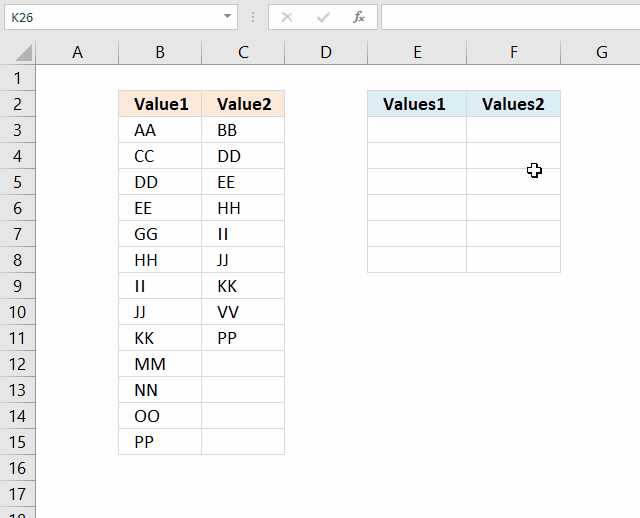1. Copy above array formula (Ctrl + c).
2. Select cell E3.
3. Press with left mouse button on in the formula bar.
4. Paste array formula (Ctrl + v) to the formula bar.
5. Press and hold CTRL + SHIFT simultaneously.
6. Press Enter once.
7. Release all keys.

The formula is now an array formula. See the curly brackets, they tell you it is an array formula. Don't enter the curly brackets yourself, they appear if you enter it correctly, like this:

{=INDEX(\$A\$2:\$A\$14, SMALL(IF(COUNTIF(\$B\$2:\$B\$10, \$A\$2:\$A\$14)=0, MATCH(ROW(\$A\$2:\$A\$14), ROW(\$A\$2:\$A\$14)), ""), ROW(A1)))}

#### How to copy array formula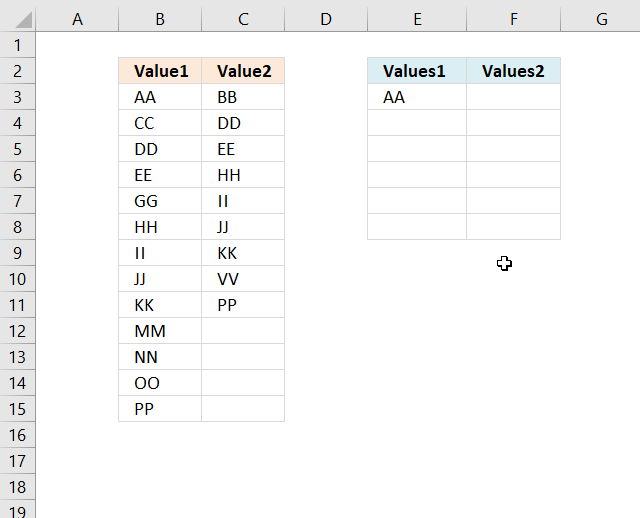1. Select cell E3.
2. Copy (Ctrl + c).
3. Select cell range E4:E8.
4. Paste (Ctrl + v).

### Explaining array formula in cell E3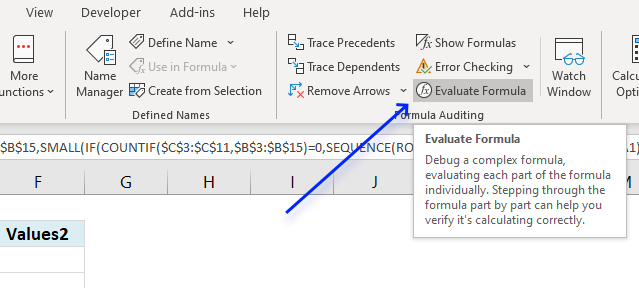I recommend the "Evaluate Formula" tool when you want to understand, troubleshoot or examine a specific formula.

Select the cell containing the formula you want to evaluate. Go to tab "Formulas" on the ribbon, press with left mouse button on the "Evaluate Formula" button, see image above.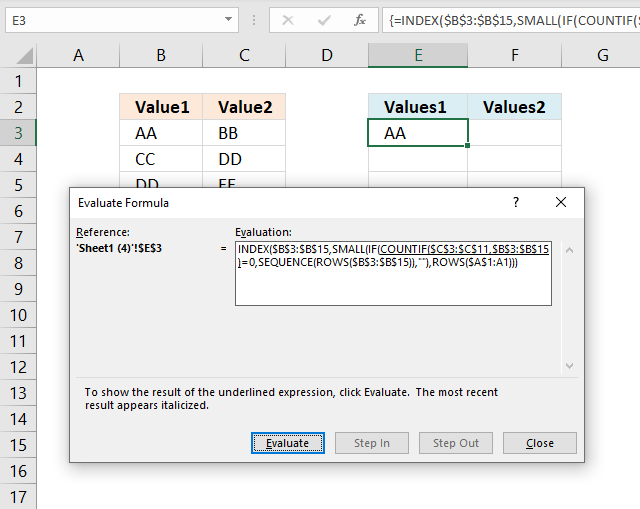A dialog box appears, it shows the formula and the button "Evaluate" below the formula allows you to go through the formula calculations step by step.

##### Step 1 - Count values in column C based on values in column B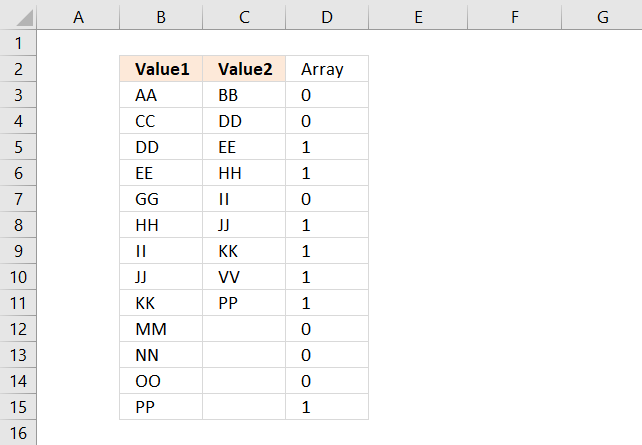The COUNTIF function lets you count values based on a condition, however, it is also possible to use multiple conditions but then the function returns an array of values instead of a single value.

This is what makes the formula an array formula. Here are the arguments in the COUNTIF function:

COUNTIF(rangecriteria)

COUNTIF(\$C\$3:\$C\$11, \$B\$3:\$B\$15)

becomes

COUNTIF({"BB"; "DD"; "EE"; "HH"; "II"; "JJ"; "KK"; "VV"; "PP"}, \$B\$3:\$B\$15)

becomes

COUNTIF({"BB"; "DD"; "EE"; "HH"; "II"; "JJ"; "KK"; "VV"; "PP"}, {"AA"; "CC"; "DD"; "EE"; "GG"; "HH"; "II"; "JJ"; "KK"; "MM"; "NN"; "OO"; "PP"})

and returns the following array of values:

{0; 0; 1; 1; 0; 1; 1; 1; 1; 0; 0; 0; 1}

The position of each value in the array is very important, they make it possible to identify and extract the values we want. The position of each value in the array corresponds to the value in column B, see image above.

A 0 (zero) means that the value in column B is not found in column C. 1 is that the value in column B is found once in column C.

##### Step 2 - Check if they are equal to 0 (zero)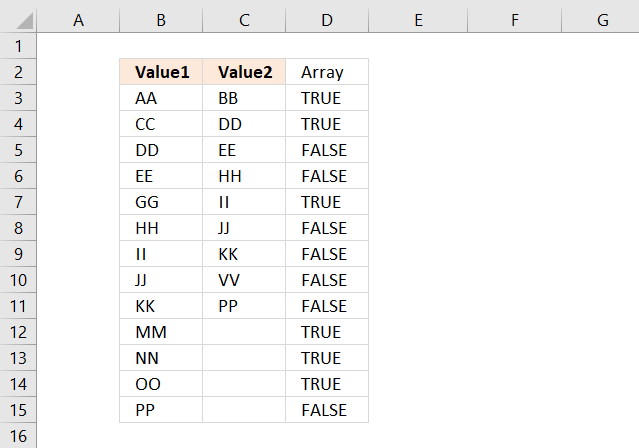The equal sign checks if the values are equal to 0 (zero) and returns the boolean values TRUE or FALSE.

COUNTIF(\$C\$3:\$C\$11, \$B\$3:\$B\$15)=0

becomes

{0; 0; 1; 1; 0; 1; 1; 1; 1; 0; 0; 0; 1}=0

and returns

{TRUE; TRUE; FALSE; FALSE; TRUE; FALSE; FALSE; FALSE; FALSE; TRUE; TRUE; TRUE; FALSE}

##### Step 3 - If they are equal to zero, return the corresponding relative row number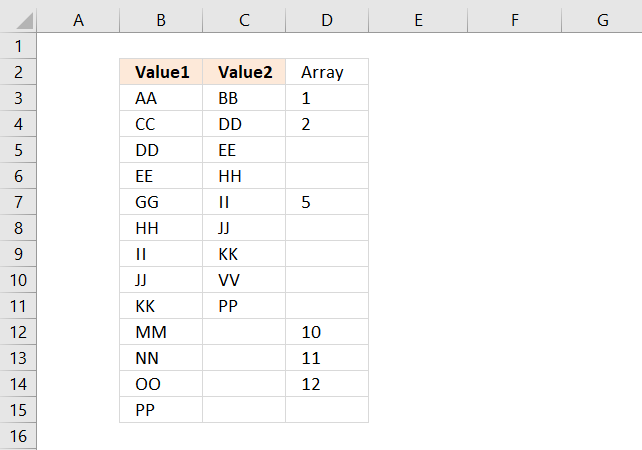The IF function allows you to return a specific value if the logical test is TRUE and another value if FALSE.

IF(logical_test, [value_if_true], [value_if_false])

IF(COUNTIF(\$C\$3:\$C\$11, \$B\$3:\$B\$15)=0, MATCH(ROW(\$B\$3:\$B\$15), ROW(\$B\$3:\$B\$15)), "")

becomes

IF({TRUE; TRUE; FALSE; FALSE; TRUE; FALSE; FALSE; FALSE; FALSE; TRUE; TRUE; TRUE; FALSE}, MATCH(ROW(\$B\$3:\$B\$15), ROW(\$B\$3:\$B\$15)), "")

The MATCH and ROW functions create an array from 1 to 11 which we then will use to extract the correct value from cell range B3:B15.

MATCH(ROW(\$B\$3:\$B\$15), ROW(\$B\$3:\$B\$15))

becomes

MATCH({3; 4; 5; 6; 7; 8; 9; 10; 11; 12; 13; 14; 15}, {3; 4; 5; 6; 7; 8; 9; 10; 11; 12; 13; 14; 15})

and returns

{1; 2; 3; 4; 5; 6; 7; 8; 9; 10; 11; 12; 13}.

##### Step 4 - Return the k-th smallest row number

The SMALL function returns the k-th smallest number from an array or cell range.

SMALL(IF(COUNTIF(\$C\$3:\$C\$11, \$B\$3:\$B\$15)=0, MATCH(ROW(\$B\$3:\$B\$15), ROW(\$B\$3:\$B\$15)), ""), ROWS(\$A\$1:A1))

beomes

SMALL({1; 2; 3; 4; 5; 6; 7; 8; 9; 10; 11; 12; 13}, ROWS(\$A\$1:A1))

The ROWS function counts the number of rows in a given cell reference. The cell ref in this example expands when you copy the cell and paste to cells below. This makes the SMALL function return a new number in each cell.

SMALL({1; 2; 3; 4; 5; 6; 7; 8; 9; 10; 11; 12; 13}, ROWS(\$A\$1:A1))

becomes

SMALL({1; 2; 3; 4; 5; 6; 7; 8; 9; 10; 11; 12; 13}, 1)

and returns 1.

##### Step 5 - Return value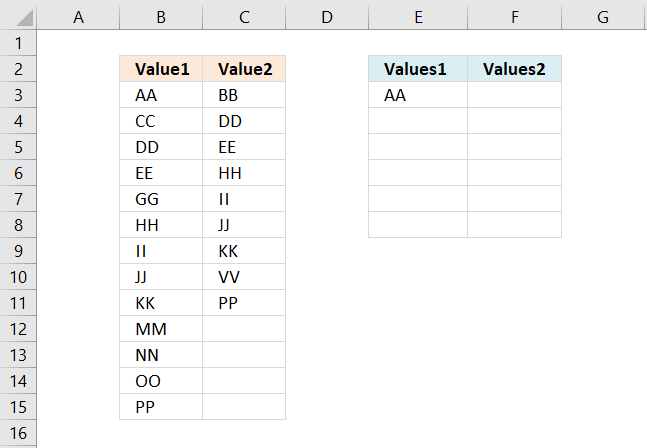The INDEX function returns a value or multiple values based on a row and/or column number.

INDEX(\$B\$3:\$B\$15, SMALL(IF(COUNTIF(\$C\$3:\$C\$11, \$B\$3:\$B\$15)=0, MATCH(ROW(\$B\$3:\$B\$15), ROW(\$B\$3:\$B\$15)), ""), ROWS(\$A\$1:A1)))

becomes

INDEX(\$B\$3:\$B\$15, 1)

and returns "AA" in cell E3.

### Recommended articles